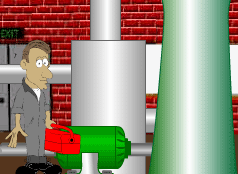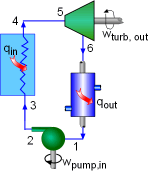Ch 10. Rankine Cycle Multimedia Engineering Thermodynamics RankineCycle Reheat Regeneration Cogeneration
 Chapter 1. Basics 2. Pure Substances 3. First Law 4. Energy Analysis 5. Second Law 6. Entropy 7. Exergy Analysis 8. Gas Power Cyc 9. Brayton Cycle 10. Rankine Cycle Appendix Basic Math Units Thermo Tables Search eBooks Dynamics Fluids Math Mechanics Statics Thermodynamics Author(s): Meirong Huang Kurt Gramoll ©Kurt GramollTHERMODYNAMICS - CASE STUDY IntroductionProblem DescriptionThe Actual Cycle Neon, who works in a steam power plant, is asked to monitor the performance of a new system. The new system is running a Rankine cycle with water as the working fluid. What is known: The Schematic of the new system is shown on the left. The design data is: Condenser Pressure: 10 kPa Boiler Pressure: 16 MPa Steam temperature at the turbine inlet: 600oC The actual data measured by Neon are: P1 = 9 kPa       T1 = 38oC P2 = 16 MPa     P3 = 15.9 MPa P4 = 15.2 MPa  T4 = 625oC P5 = 15 MPa     T5 = 600oC P6 = 10 kPa    The pump has an efficiency of 85% and the turbine has an efficiency of 80%.    The mass flow rate is 20 kg/s. Question VALIGN="TOP"> What is the net work output? What is the thermal efficiency of the cycle? What is the thermal efficiency if modeling the cycle as an ideal Rankine cycle with the design data? Approach Model the cycle of new system as a Rankine cycle. Use the water table to determine the enthalpy at each state. The net work output equals the difference between the turbine work and the pump work. The heat input equals the enthalpy difference between the boiler exit and inlet.